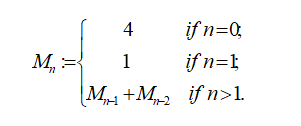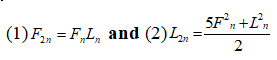# The Mulatu Numbers in Actions

• Mulatu Lemma Professor
• Latrice Tanksley
• Keisha Brown
Keywords: Mulatu numbers, Mulatu sequences, Fibonacci numbers, Lucas numbers, Fibonacci sequences, Lucas sequences

### Abstract

The Mulatu numbers were introduced in . The numbers are sequences of numbers of the form: 4,1, 5,6,11,17,28,45...  The numbers have wonderful and amazing properties and patterns.

In mathematical terms, the sequence of the Mulatu numbers is defined by the following recurrence relation:The double Angel Formulas for Fibonacci and Lucas numbers are given by the following formulas respectively.Since both the Fibonacci and Lucas numbers have double angle Formulas, It is natural to ask if such formula exists for Mulatu Numbers. The answer is affirmative and produces the following paper.

2000 Mathematical Subject Classification:  11

### Author Biographies

Mulatu Lemma, Professor

Department of Mathematics

College of Science and Technology

Savannah State University USA

Latrice Tanksley

Department of Mathematics

Savannah State University

Savannah, Georgia

The USA.

Keisha Brown

Department of Mathematics

Savannah state University

Savannah, Georgia

USA

### References

Mulatu Lemma, The Mulatu Numbers, Advances and Applications in Mathematical Sciences, Volume 10, issue 4,august 2011, page 431-440.
Burton, D. M., Elementary number theory. New York City, New York: McGraw-Hill. 1998.
Published
2021-02-22
How to Cite
Lemma, M., Tanksley, L., & Brown, K. (2021). The Mulatu Numbers in Actions. GPH - International Journal of Mathematics, 4(02), 11-16. Retrieved from http://gphjournal.org/index.php/m/article/view/377
Section
Articles

1 2 > >>